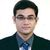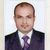# QlikView App Dev

Discussion Board for collaboration related to QlikView App Development.

Announcements
Our next Qlik Insider session will cover new key capabilities. Join us August 11th REGISTER TODAY
cancel
Showing results for
Did you mean:Contributor II

## Range of years

Hi,

I want to display data from 5 years back to the current year. Therefore, I do not want the expression to be fixed in the sense that it shows 2012-2017 because next year I want it to show 2013-2018. Is there a variable I could create (I already have a variable vCurrentYear) or an expression I could use to show a range of dates?

Right now, this is the portion of the expression and it only shows the data point for 2017 because of vCurrentYear:

Sum({\$<Year=(\$(vCurrentYear))>}[Revenue Amt]/1000))

4 RepliesLuminary Alumni

Hi,

Instead you can create a variable which stores the minimum year dynamically and then use it like below in expression.

Sum({\$<Year={">=\$(vMinYear)"}>}[Revenue Amt]/1000)

Regards,

Kaushik Solanki

Regards,
KaushikMVP

Try this

Sum({\$<Year=("\$(='>=' & (Year(Today()) - 4) & '<=' & Year(Today()))"}>} [Revenue Amt]/1000)

When you put this in a text box object (or KPI object in Qlik Sense), you should see this

>=2013<=2017MVP

Another way of doing the same is this expression calculates the Revenue from current year to the last 5 years with addmonths to -60 months dynamically.

=Sum({<Year = {">=\$(=vMinYear)   <=\$(=Year(Addmonths(Today(),-60))) "} >} [Revenue Amt]/1000 )MVP

Or simply like this:

=Sum({\$<Year = ('>=\$(=\$(vCurrentYear)-4)'}>} [Revenue Amt]/1000)

The blue equal sign in the \$-sign expansion forces the content to be treated as an expression. If your variable vCurrentYear is correctly set, the calculation will result in a value indicating four years ago.Community Browser